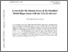# A search for the dimuon decay of the Standard Model Higgs boson with the ATLAS detector

Collaboration, ATLAS
A search for the dimuon decay of the Standard Model Higgs boson with the ATLAS detector. Phys. Lett. B, 812. 135980 - ?.Text 2007.07830v1.pdf - Submitted version Download (1MB) | Preview

## Abstract

A search for the dimuon decay of the Standard Model (SM) Higgs boson is performed using data corresponding to an integrated luminosity of 139 fb$^{-1}$ collected with the ATLAS detector in Run2 $pp$ collisions at $\sqrt{s}=13$ TeV at the Large Hadron Collider. The observed (expected) significance over the background-only hypothesis for a Higgs boson with a mass of 125.09 GeV is $2.0\,\sigma$ ($1.7\,\sigma$). The observed upper limit on the cross section times branching ratio for $pp \to H \to \mu\mu$ is 2.2 times the SM prediction at 95% confidence level, while the expected limit on a $H \to \mu\mu$ signal assuming the absence (presence) of a SM signal is $1.1$ ($2.0$). The best-fit value of the signal strength parameter, defined as the ratio of the observed signal yield to the one expected in the SM, is $\mu = 1.2 \pm 0.6$.

Item Type: Article 44 pages in total, author list starting page 28, 2 figures, 3 tables, Published by Phys. Lett. B. All figures including auxiliary figures are available at https://atlas.web.cern.ch/Atlas/GROUPS/PHYSICS/PAPERS/HIGG-2019-14 hep-ex, hep-ex Symplectic Admin 15 Dec 2020 10:36 18 Jan 2023 23:18 10.1016/j.physletb.2020.135980 https://livrepository.liverpool.ac.uk/id/eprint/3110208